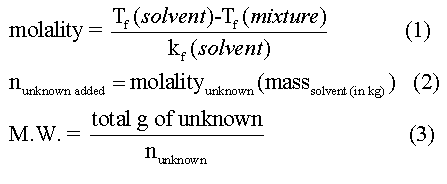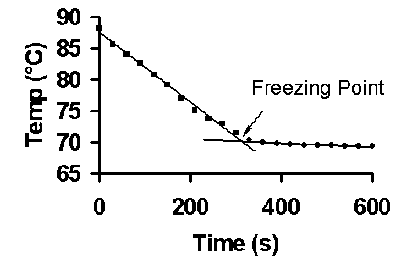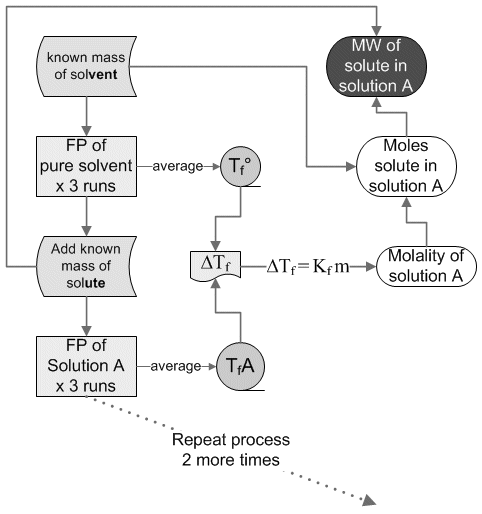Molar Mass Determination from Freezing Point Depression

This is a new procedure. The purpose of implementing this procedure is to reduce waste and use less toxic materials. Previously, compounds such as p-dichlorobenzene, biphenyl, benzil, and naphthalene were used. As these compounds are toxic and therefore potentially hazardous, they have been replaced with naturally occurring non-toxic fatty acids. One of the green objectives of this lab is to reduce waste by converting the used fatty acids into useful products such as soap, candles or bio-diesel fuel. For this reason, waste disposal directions should be followed carefully.

When a nonvolatile solute is dissolved in a solvent, the solvent’s boiling point is increased, its freezing point is decreased, and its osmotic pressure is increased. These characteristics of a solution are termed the colligative properties. Colligative effects are observed in many phenomena such as the melting of ice on winter roads from “salting”, addition of antifreeze to a car’s radiator to prevent “freeze up”, and the rising of water in trees, due to osmotic pressure.

The amount the properties of a mixture change due to colligative effects depends on the number of moles of solute particles dissolved in the solvent and not the nature of the particles. It is therefore imperative to know whether the dissolved solute produces one mole of particles for every mole dissolved, or whether the solute dissociates into two, three or more particles on dissolving. Ionic compounds when dissolved in water produce more than one mole of particles (ions). NaCl produces two moles of ions, while CaCl2 produces three moles of particles.

The relationship between the boiling point elevation (BPE) and the freezing point depression (FPD) to the corresponding change in temperature is given by the following equations:
BPE :   ΔTb = ikbm                            FPD :   ΔTf = ikf m
Where,
ΔTb is the difference between the boiling point of the solution and the boiling point of the solvent and  DTf is the difference between the freezing point of the solution and the freezing point of the solvent. The change is temperature,  ΔT, is always a positive value. The number of particles is represented by the Van’t Hoff Factor, i. In this experiment the solute is molecular and i will equal one. The concentration of the solution is determined in molality, m. The molal boiling point constant, kb, and the molal freezing point constant, kf, are specific for each solvent. Some representative values are shown below.

Solvent                kb (°C kg/ mole)           kf (°C kg/ mole)
water                         0.52                            1.86
benzene                     2.53                            5.10
camphor                    40.0                            5.95
stearic acid                                                   4.50

One use of colligative properties is the determination of the molecular weight of a substance. In this experiment, the freezing point of pure stearic acid will be measured. A weighed quantity of an unidentified sample will be added to the pure stearic acid and the freezing point re-determined. The difference between the freezing points of pure stearic acid and the mixture is the freezing point depression, ΔTf. Knowing the molal freezing point constant for stearic acid, the molecular weight of the unidentified sample can be determined. by using the following equations.Data Treatment

To accurately determine the freezing points of pure stearic acid and each of the solutions, “cooling curves” of temperature (y-axis) versus time (x-axis) are plotted. Data where the temperature changes by more than 0.5 °C per 30 seconds is plotted as one series and data where the temperature changes by less than 0.5 °C per 30 seconds as a second series. The freezing point is obtained by finding the intersection of the two best fit lines, see Figure B. This can be done on the fly by simply monitoring the temperature as it drops.Figure B. Example of colligative properties data obtained using a mixture of stearic and myristic acids. The figure illustrates the data analysis process for determining the freezing point of the mixture.

Procedure (sortof):
You will place ~5g of pure stearic acid in a test tube, melt it and determine it's MP. You will then add ~0.3 g of solute and measure the MP of this new solution (x3 for good measure of course). You will then add another ~0.1 g and repeat the MP determination again (x3) and again...

Report: Short form memo  You are ultimately trying to determine the molecular weight and identity of your unidentified sample using the list below.
 Myristic  Acid C14H28O2 Palmitic Acid C16H32O2 Lauric Acid C12H24O2

Before you enter the lab, you must show the instructor a piece of recycled paper with places for ALL the data you will be taking that day. If you do not have this, you will not be doing the lab that day.

Lab picture:Prelab questions:

1.        A researcher takes 5.00 g of tridecanoic acid (MW = 214.34 g / mole) and dissolves it in 20.0 g of stearic acid (kf = 4.5 °C kg / mole). What is the observed change in the freezing point from the pure stearic acid to the stearic acid with tridecanoic acid added? Assume the compound is molecular i.e. cannot dissociate.

2. Do colligative properties depend on the number of particles dissolved, the identity of the particles dissolved, or both?

3. Give a brief summary of the hazards of stearic acid from the MSDS.

01.31.07 07:29